# Volume of a Pyramid

Volume of a Pyramid• The volume of a pyramid is found by multiplying the area of the base by the height of the pyramid and then dividing by 3.
• The area of the base tells us the name of the pyramid.
• The formula for the volume of a pyramid is given as Volume = Base Area × Height ÷ 3.
• Volume is measured in units cubed.
• If the sides are measured in cm, then the volume is measured in cm3 and if the sides are measured in m, the volume is measured in m3.

To find the volume of a pyramid, multiply its base area by its height and then divide by 3.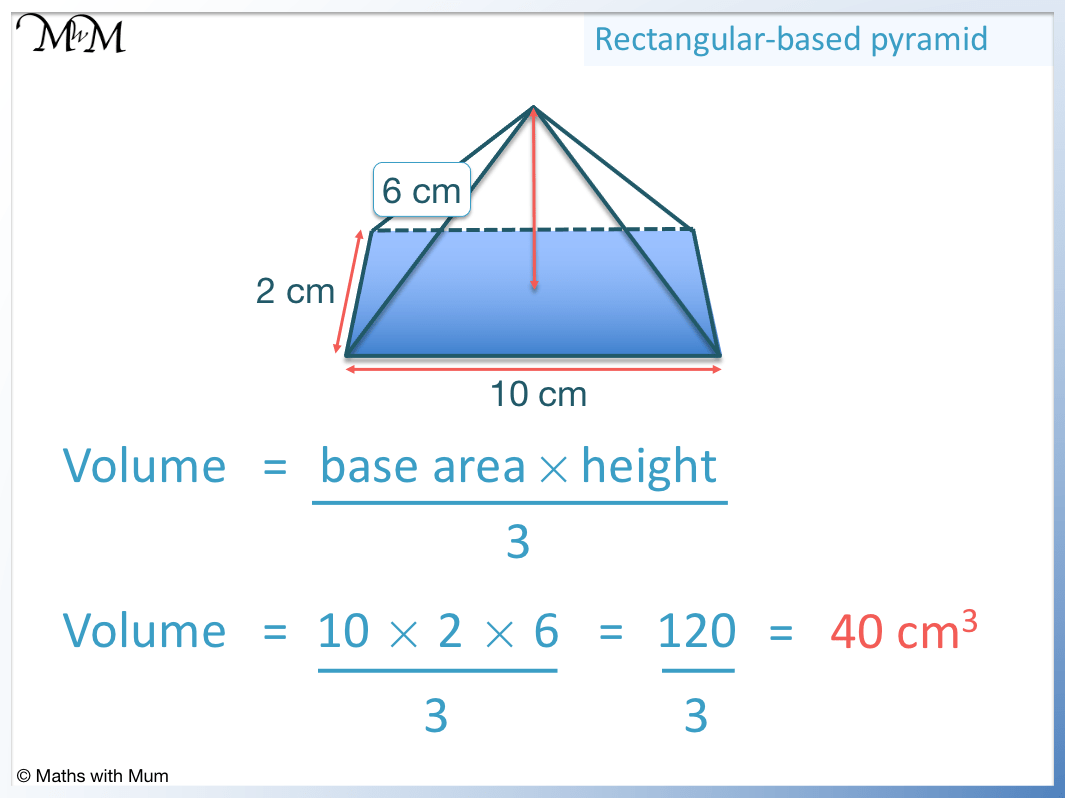• This pyramid has a rectangular base and so, it is called a rectangular-based pyramid.
• To find the volume, multiply the area of the base by the height of the pyramid and divide by 3.
• The area of the rectangular base is length × width.
• The area of the base is 10 × 2 = 20 cm2.
• We multiply this area by the height and divide by 3.
• The height is 6. So 20 × 6 = 120 and 120 ÷ 3 = 40.
• The volume of this rectangular-based pyramid is 40 cm3.# Volume of a Pyramid

## How to Find the Volume of a Pyramid

To find the volume of a pyramid, multiply the base area by the height and divide this by three. The volume of a pyramid is found with the formula V = B × h ÷ 3, where B is the base area and h is the height of the pyramid.

The base area depends on the shape of the base. No matter what the base, the volume of a pyramid is found by multiplying the area of the base by the height of the pyramid and then dividing by 3.For example for a triangular-based pyramid with a base area of 10 cm2 and a height of 6 cm, the volume is found by multiplying the area by the height and dividing by 3. 10 × 6 ÷ 3 = 20 and so, the volume of the pyramid is 20 cm3.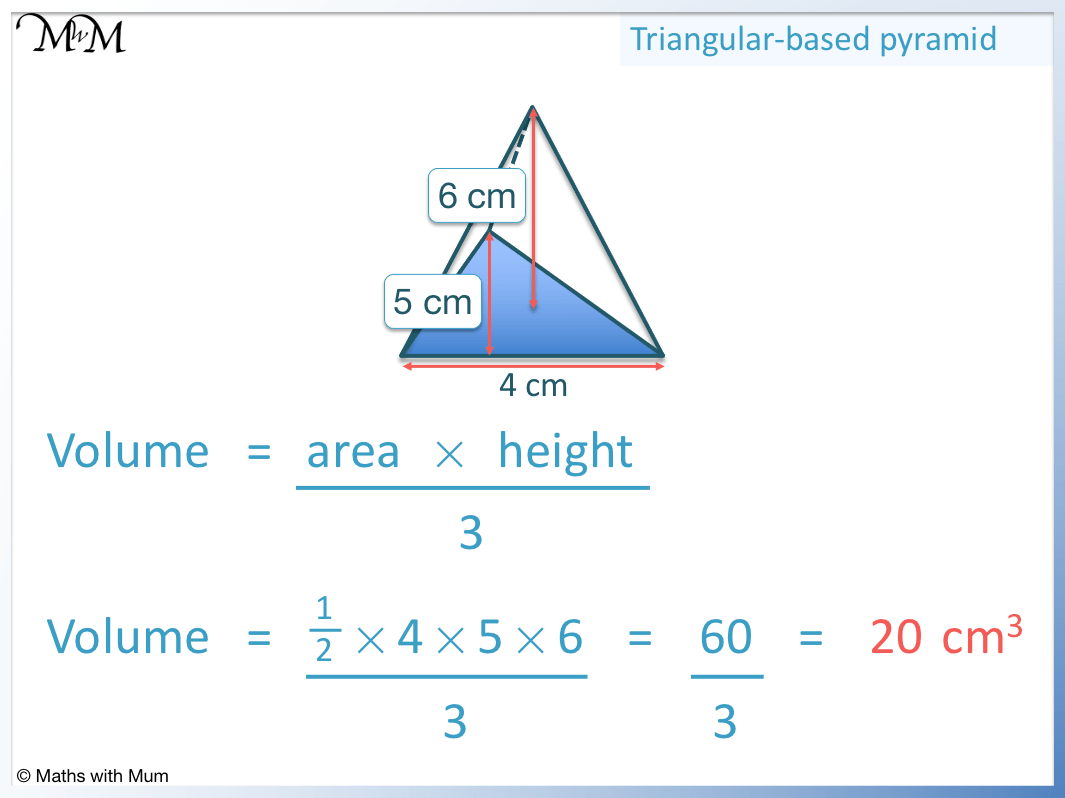The base is a triangle and the area of a triangle is 1/2 × base × height. The base of the triangle is 4 cm and the height of the triangle is 5 cm. The height of the triangle is at right angles to the base of the triangle and it is different to the height of the pyramid, which is 6 cm.

Area = 1/2 × base × height and so Area = 1/2 × 4 × 5, which equals 10 cm2.

Once the base area has been found, multiply this by the height of the pyramid and divide by 3 to find the volume.

10 × 6 ÷ 3 = 20 and so, the volume is 20 cm3.

## Volume of a Rectangular-Based Pyramid

To find the volume of a rectangular-based pyramid, multiply the area of the rectangular base by the height of the pyramid and divide by 3. The volume of a rectangular-based pyramid is found by the formula V = lwh ÷ 3, where l is the length of the rectangle, w is the width of the rectangle and h is the height of the pyramid.

The area of a rectangle is length × width. The area of the rectangular base is multiplied by the height of the pyramid and then divided by 3.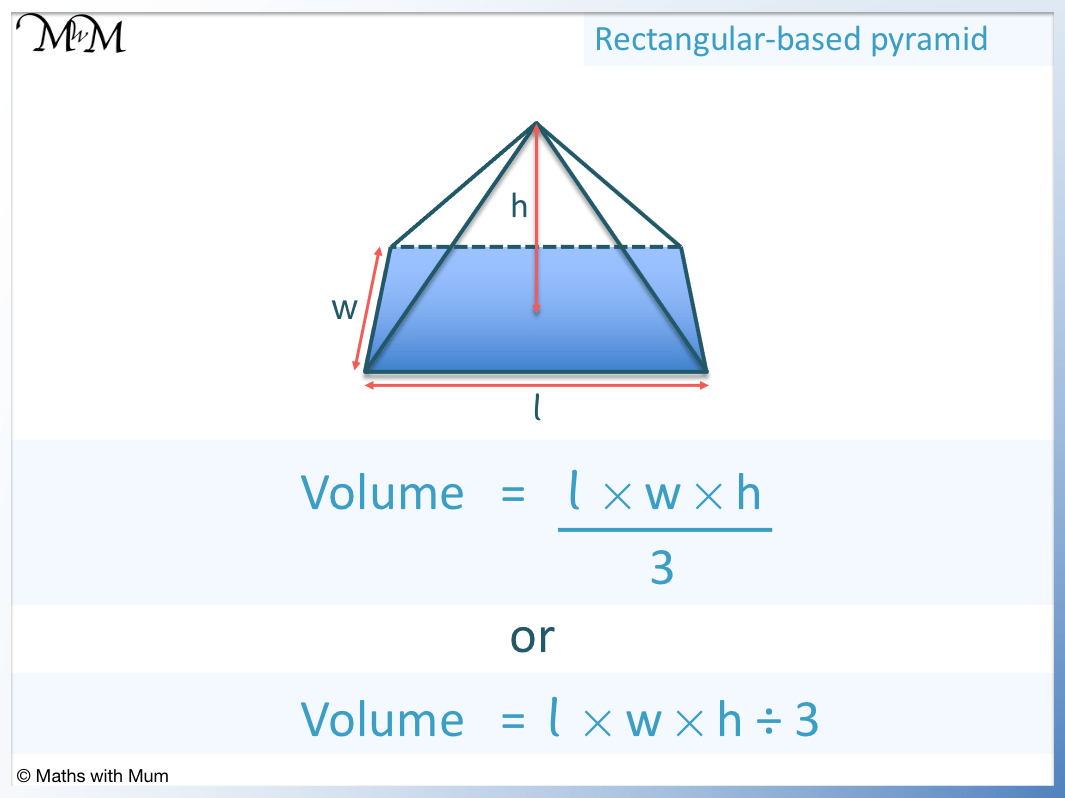The formula for the volume of a rectangular-based pyramid can be written as V = l × w × h/3 or as V = l × w × h ÷ 3.

For example, here is a rectangular pyramid with base length of 10 cm, base width of 2 cm and a height of 6 cm.The volume is found by multiplying the area of the base by the height of the pyramid and dividing by 3.

The area of a rectangle is length × width, which in this example is 10 × 2. The area of the rectangular base is 20 cm2/.

To find the volume, multiply this area by the height and divide by 3.

20 × 6 ÷ 3 = 40 and so, the volume of the pyramid is 40 cm3.

## Volume of a Square-Based Pyramid

To find the volume of a square-based pyramid, multiply the area of the square base by the height of the pyramid and divide this by 3. The volume of a square-based pyramid is found with the formula V = l2 × h ÷ 3, where l is the length of the side of the square base and h is the height of the pyramid.

The side lengths of a square are all the same size and so, the area of the square base is simply one side multiplied by itself. For a square with side length l, the area of the square is l2.For example in a square-based pyramid with a side length of 2 cm and a height of 3 cm, the volume is found by multiplying the area of the square base by the height of the pyramid and dividing by 3.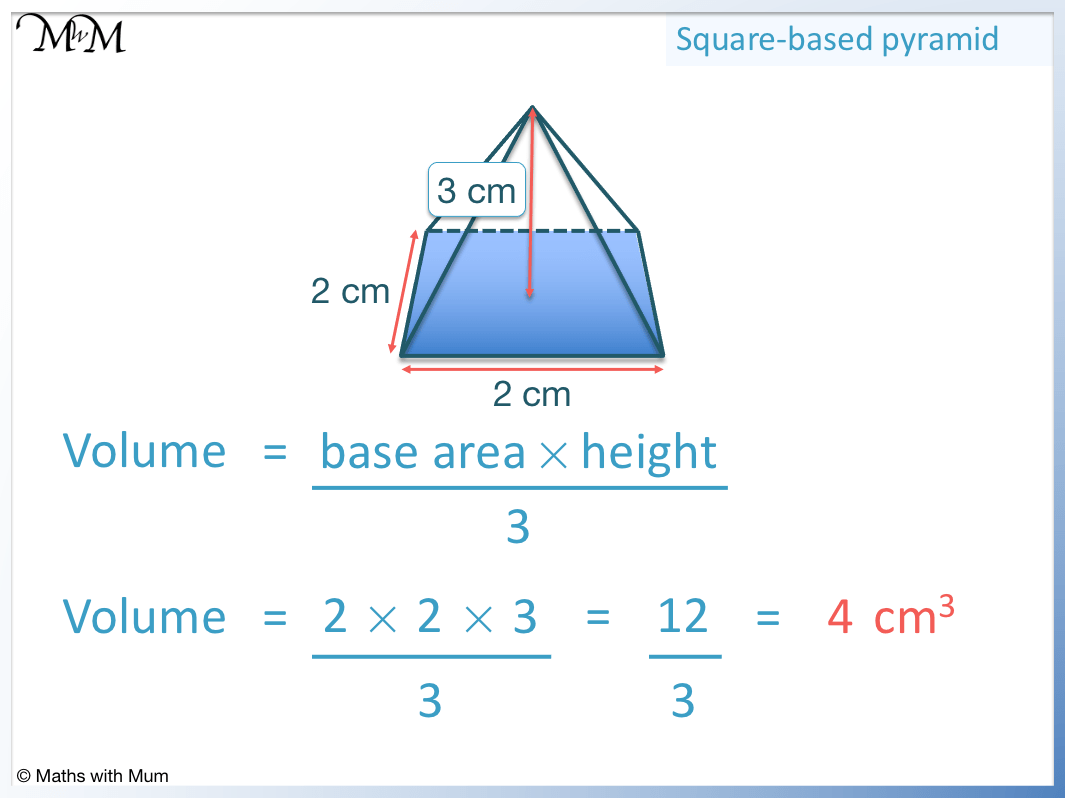The area of the square base is the length squared. We simply multiply the length of one side of the square by itself.

2 × 2 = 4 and so, the area of the base is 4 cm2.

To find the volume, we multiply the area of this base by the height and divide by 3.

The height is 3, so the volume is 4 × 3 ÷ 3. The volume is 4 cm3.

## Volume of a Triangular-Based Pyramid

To find the volume of a triangular-based pyramid, multiply the area of the trianglular base by the height of the pyramid and divide this by 3. The volume of a triangular-based pyramid is found with the formula: Volume = 1/3 × Base Area × Height, where the base area is 1/2 × Triangle Base × Triangle Height.The base area of a triangular pyramid is a triangle and the area of a triangle is 1/2 × Triangle Base × Triangle Height.

In this example, we will call the height of the triangular base ‘h’ and the height of the pyramid ‘H’.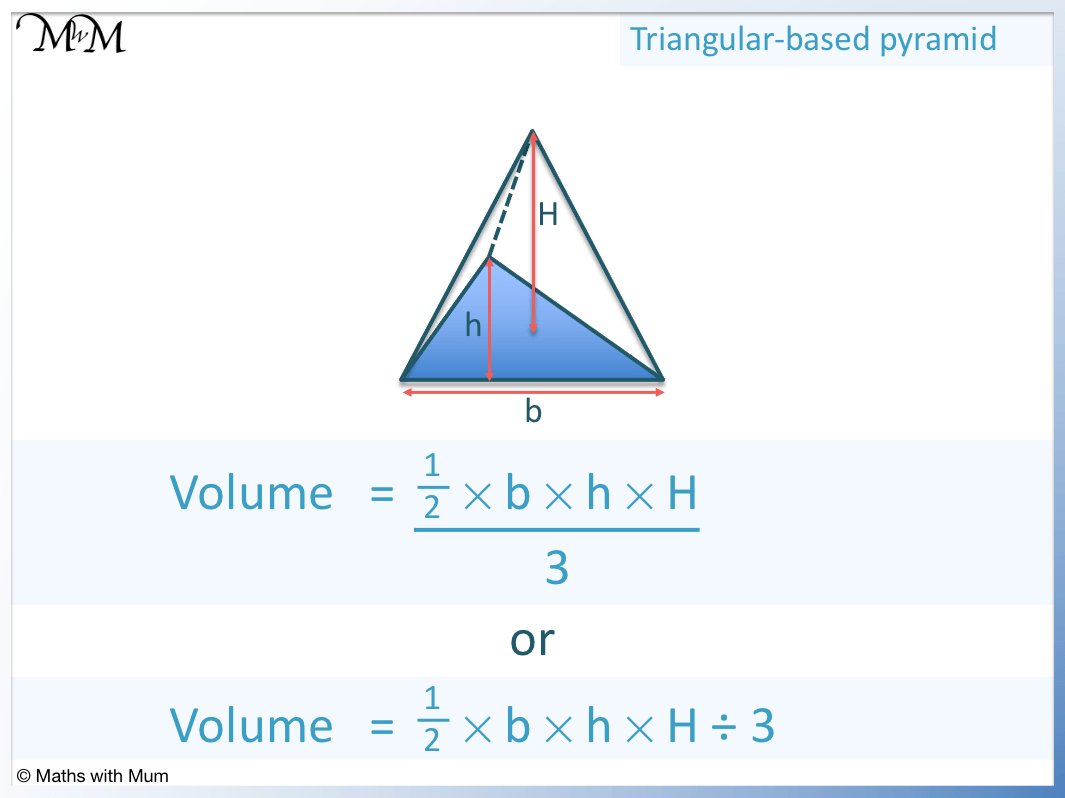V = 1/2 × b × h × H ÷ 3.

For example, in a triangular pyramid with triangle base of 4 cm, triangle height of 5 cm and pyramid height of 6 cm, the volume is found by V = 1/2 × 4 × 5 × 6 ÷ 3. The volume is 20 cm3.The volume of a triangular-based pyramid can be written most simply as V = bhH ÷ 6, where b is the base of the triangle, h is the height of the triangle and H is the height of the pyramid.

In this example, b = 4, h = 5 and H = 6.

V = bhH ÷ 6 and so, V = 4 × 5 × 6 ÷ 6.

V = 20 cm3.Now try our lesson on Radius and Diameter where we learn how to identify the radius and diameter of a circle.